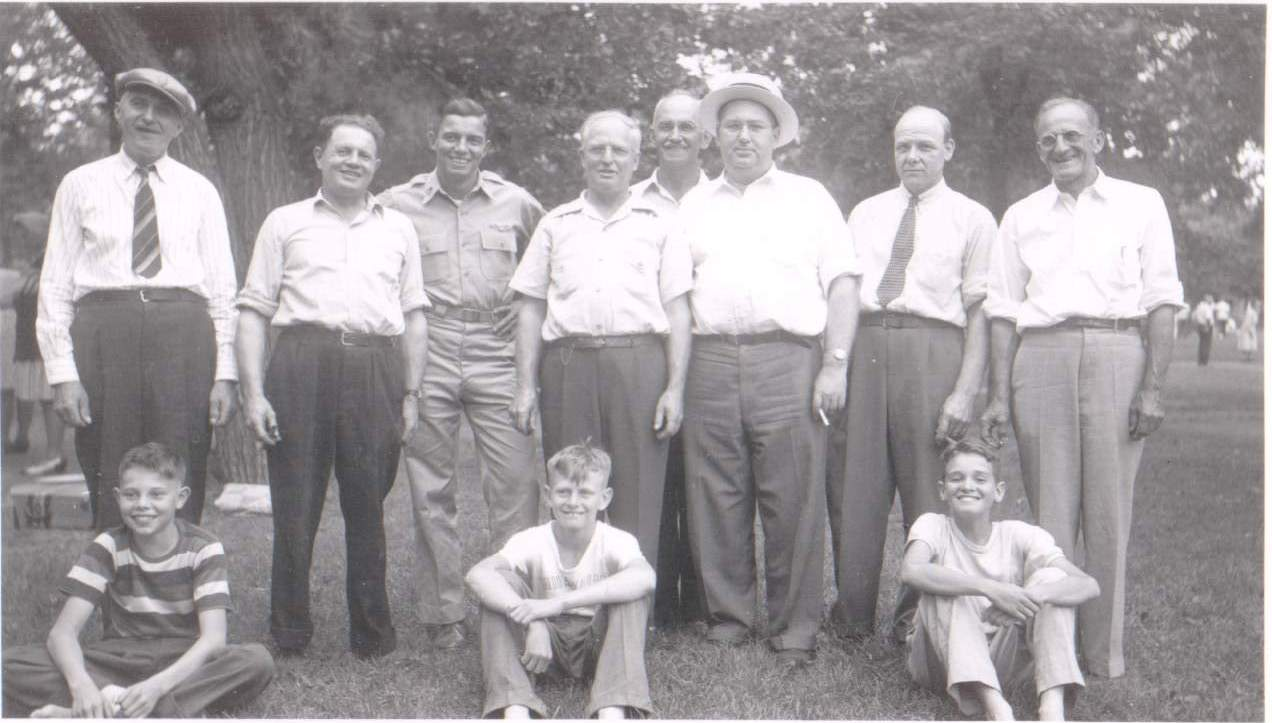## Picnic

Place mouse over face for identification of personDescription Picnic Date / Time 1942-00-00 Country / State / County / City / Placename USA, MN, xx, Melrose Persons (t-b,l-r) UNK1, Frank W Helsper, John W Dulin, John Waldorf, Noah Louis Goulah, John T Gearty, William (Bill) Gieske, UNK2, Francis (Frank) William Helsper, Calistus Elhert, Jerome James Goulah
```lib card:
dc:
title 		=	Picnic
creator 	=	Jerome Goulet
subject 	=
description 	=	Original (scanned analog or digital), names to faces, stored to LibCard "format", within image exif:
publisher 	=	Jerome Goulet
contributor 	=
date 		=	1942-00-00
resourcetype 	=
iformat 	=
if_mime 	=	image/jpeg
if_resolution 	=	1x1
if_dimensions 	=
if_dim_x 	=	1272
if_dim_y 	=	723
resourceID 	=
source 		=	photograph (analog or digital)
language 	=	eng
rights 		=	Copyright 2020, Jerome Goulet<jtgoulet@yahoo.com>, GNU General Public License
lc:
when:
dateUTC		=	1942-00-00
timeUTC		=	0000
tz		=	+0000

dateLocal	=	1942-00-00
timeLocal	=	0000
where:
admin0		=	USA
admin1		=	MN
admin2		=	xx
admin3		=	Melrose
pName		=	x
lat		=	x
lon		=	x
who:
person:
name:
surName		=	UNK1
givenName	=
aka		=
relation	=
pip:	(person in picture)
boxX		=	122
boxY		=	89
boxWidth	=	63
boxHeight	=	63
label		=
uri		=
person:
name:
surName		=	Helsper
givenName	=	Frank W
aka		=	FWH1899
relation	=
pip:	(person in picture)
boxX		=	324
boxY		=	130
boxWidth	=	60
boxHeight	=	60
label		=
uri		=
person:
name:
surName		=	Dulin
givenName	=	John W
aka		=	JWD
relation	=
pip:	(person in picture)
boxX		=	432
boxY		=	115
boxWidth	=	61
boxHeight	=	61
label		=
uri		=
person:
name:
surName		=	Waldorf
givenName	=	John
aka		=	JW
relation	=
pip:	(person in picture)
boxX		=	578
boxY		=	126
boxWidth	=	59
boxHeight	=	59
label		=
uri		=
person:
name:
surName		=	Goulah
givenName	=	Noah Louis
aka		=	NLG1893
relation	=
pip:	(person in picture)
boxX		=	645
boxY		=	104
boxWidth	=	60
boxHeight	=	60
label		=
uri		=
person:
name:
surName		=	Gearty
givenName	=	John T
aka		=	JTG1901
relation	=
pip:	(person in picture)
boxX		=	712
boxY		=	101
boxWidth	=	63
boxHeight	=	63
label		=
uri		=
person:
name:
surName		=	Gieske
givenName	=	William (Bill)
aka		=	WG
relation	=
pip:	(person in picture)
boxX		=	883
boxY		=	124
boxWidth	=	61
boxHeight	=	61
label		=
uri		=
person:
name:
surName		=	UNK2
givenName	=
aka		=
relation	=
pip:	(person in picture)
boxX		=	1028
boxY		=	115
boxWidth	=	61
boxHeight	=	61
label		=
uri		=
person:
name:
surName		=	Helsper
givenName	=	Francis (Frank) William
aka		=	FWH1930
relation	=
pip:	(person in picture)
boxX		=	110
boxY		=	468
boxWidth	=	65
boxHeight	=	65
label		=
uri		=
person:
name:
surName		=	Elhert
givenName	=	Calistus
aka		=	CE
relation	=
pip:	(person in picture)
boxX		=	546
boxY		=	472
boxWidth	=	55
boxHeight	=	55
label		=
uri		=
person:
name:
surName		=	Goulah
givenName	=	Jerome James
aka		=	JJG1931
relation	=
pip:	(person in picture)
boxX		=	936
boxY		=	453
boxWidth	=	62
boxHeight	=	62
label		=
uri		=
what:
eventName	=	Picnic
```